### Background

Deep learning is very popular recently, which is based on Neural Network, an old algorithm that had degraded for years but is resurging right now. We talk about some basic concept about Neural network today, hoping supply a intuitive perspective of it.

Before beginning, I’d like to introduce you an exicting product which help those who are blind see the world. BrianPort, which is invented by Wicab, uses you tougue to see the world. Tongue array contains 400 electrodes and is connected to the glasses. The product transfers from light to electric signal. More than 80% blind persons could pass through the block during the experiments.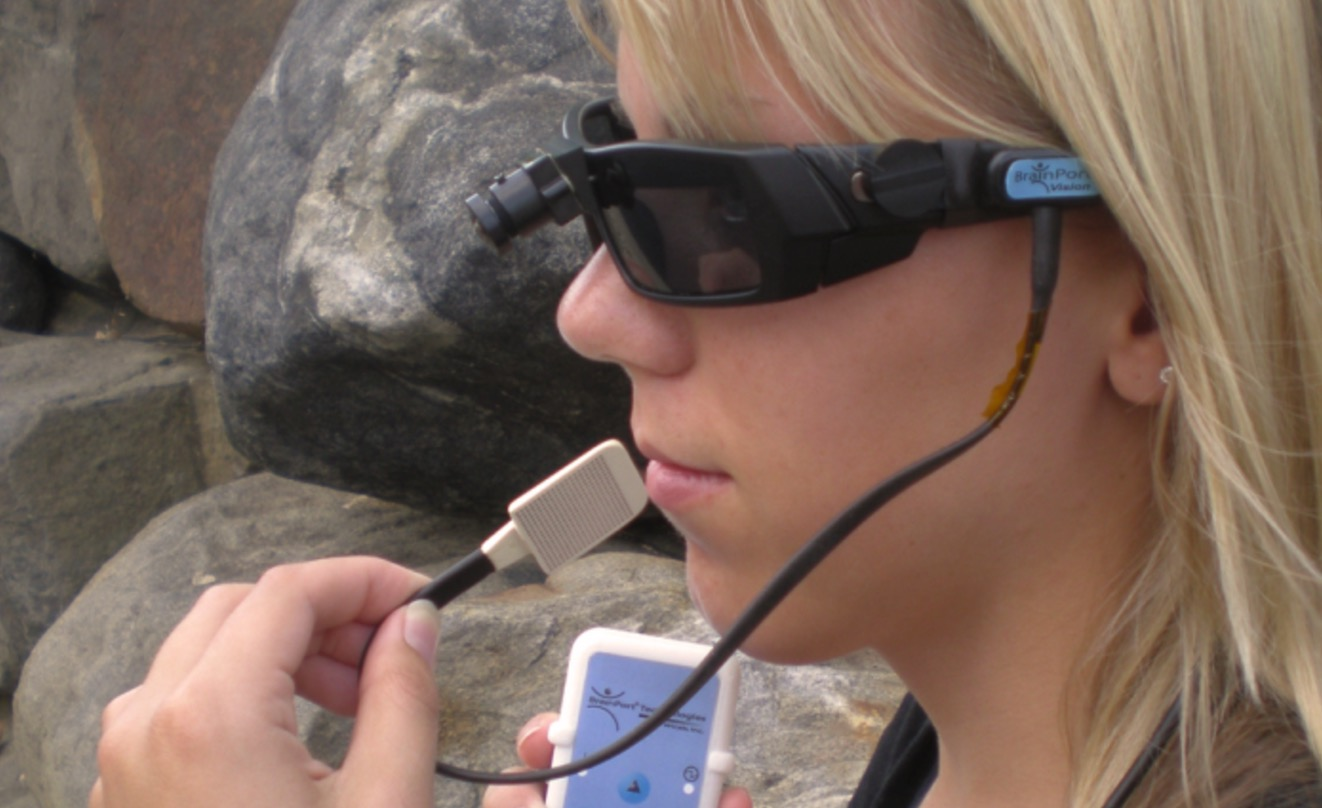In fact, Wicab takes advantage the mechanism of neural network of our brain. There are 86 billion neuron in our brain. We can smell, see, hear the world just because of these neurons. They are connect to each other to help us sense the world. Algorithm Neural Network is a way of mimic the mechanism of our brain.

### Intuition

Let’s start from the easiest model, we get $a_1$ in two steps:
step1: $z_1=w_1x_1+w_2x_2+w_3x_3$
step2: $a_1=\frac{1}{1+e^{(-z)}}$
In addition, we add a bias $w_0$ to the calculate. After letting $x_0=1$, then:
$$z=w_0x_0+w_1x_1+w_2x_2+w_3x_3$$ We always add a bias at each layer but the last to Neural Network.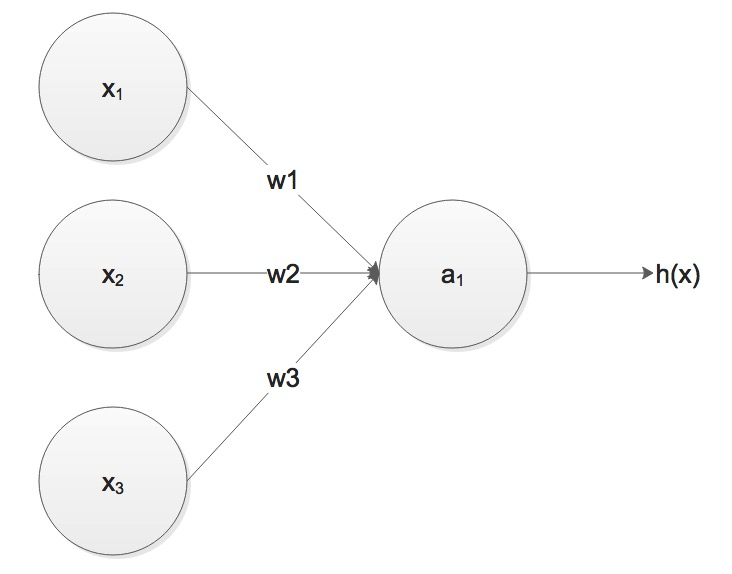If we contrast this model with logistic regression model, ww find that right now the to model is just the same: input every $x$ represents a feature. In logistic regression, we want to train a model $h_w(x)=\frac{1}{1+e^{-W^Tx}}$. The simpliest Neural Network, the model is a little complex, but if we do not take hidden layer into account, the model is just logistic regression.

### Neural Network

To approach the authentic Neural Network, we add two more nerons($a_2^{(2)}$ and $a_1^{(3)}$) to logistic regression model. Notice that the model inner green triangle box is just like logistic regression demonstrated above. There are only two layers in Logistic Regression, in contrast, we can add more layers like L2 layer. In Neural Network, we call these layers hidden layers which are neither the input(e.g. layer have $x_1, x_2, x_2$), nor the output $h(x)$. The figure below has only one hidden layer, though we can add many hidden layers to the model.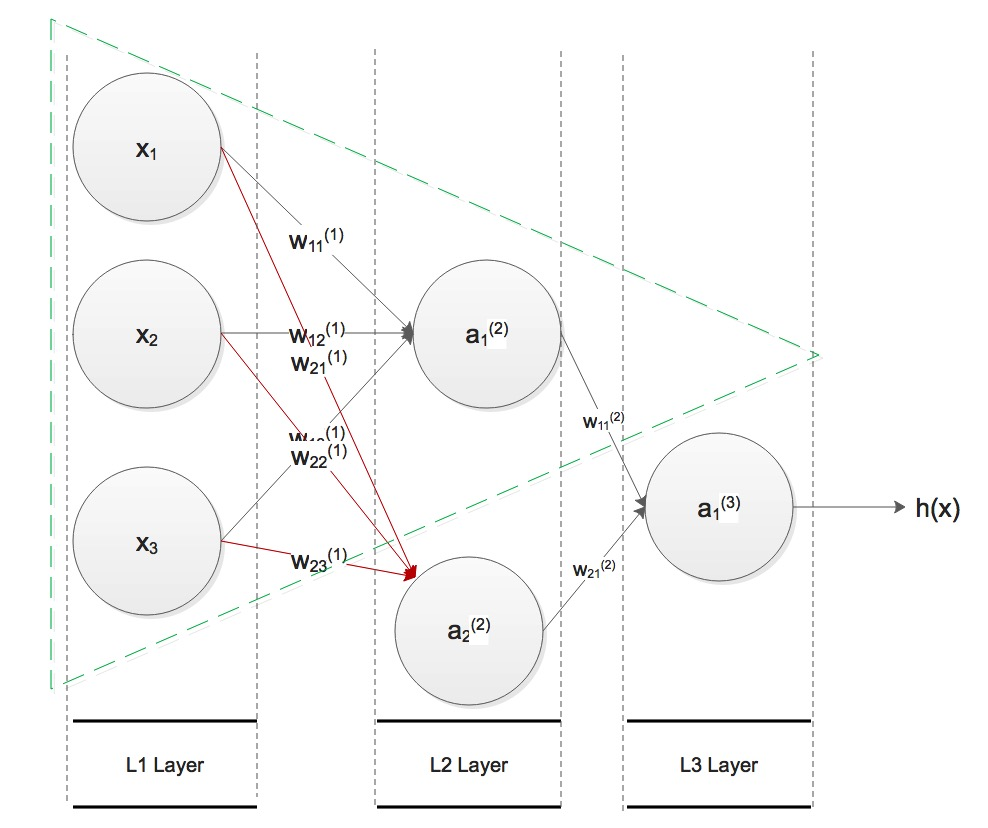Look at the figure above, let’s look at the definition of Neural Network, take $w_{12}^{(1)}$ for example, the subscript $_{12}$ represents the weight from the former layer $2nd$ unit to the current layer $1st$ unit. The superscript $^1$ represents former layer is layer L1. These $w$ are named weights of Neural Network. The sigmoid function $f=\frac{1}{1+e^{-x}}$ is activation function. We can choose other activation function such as symmetrical sigmoid $S(x)=\frac{1-e^{-x}}{1+e^{-x}}$. Now let’s think about how to calculate $h(x)$, for the L2 layer, we have:
\begin{align}
& z_1^{(2)}=w_{10}^{(1)}x_0 + w_{11}^{(1)}x_1 + w_{12}^{(1)}x_2 + w_{13}^{(1)}x_3\
& z_2^{(2)}=w_{20}^{(1)}x_0 + w_{21}^{(1)}x_1 + w_{22}^{(1)}x_2 + w_{23}^{(1)}x_3\
& a_1^{(2)} = g(w_{10}^{(1)}x_0 + w_{11}^{(1)}x_1 + w_{12}^{(1)}x_2 + w_{13}^{(1)}x_3)=g(z_1^{2})\
& a_2^{(2)} = g(w_{20}^{(1)}x_0 + w_{21}^{(1)}x_1 + w_{22}^{(1)}x_2 + w_{23}^{(1)}x_3)=g(z_2^{2})
\end{align}
Here, $g()$ is the activation function. Notice that if we use matrices represent the equation, result will be simpler:
$$a^{(2)} = g(z^{(2)}) = g(W^{(1)} a^{(1)})$$
Here, we let $a_i^{(1)}=x_i$. We can conclude one more step, for layer k, we have:
$$a^{(k)} = g(z^{(k)}) = g(W^{(k-1)} a^{(k-1)})$$
Then for the L3 Layer, we have only one neural:
\begin{align}
h(x) = a_1^{3}=g(w_{10}^{(1)}a_0^{(2)} + w_{11}^{(1)}a_1^{(2)} + w_{12}^{(1)}a_2^{(2)})=g(z_1^{3})
\end{align}
If we substitute $a_1^{2}$ and $a_2^{2}$ for elme $h(x)$, we have:
\begin{align}
h(x)=a_1^{3}=g(w_{10}^{(1)}\cdot 1 + w_{11}^{(1)}
\cdot g(z_1^{(2)})+ w_{12}^{(1)}\cdot g(z_2^{(2)}))
\end{align}
The formula show that we use $g()$ function once and once again to nest the input, and compute the output eventaully. It is rather a non-linear classifier than linear classifier such as Linear Regression and Logistic Regression.

### More Complicated Network

A Neural Network can be very complex, as long as we add more hidden layer into the network, the figure showed below is a neural network which has 20 layers, which means it has 1 input layer, 1 output layer and 18 hidden layers. From the connected weight we can imagine how much many weight we would calculate if we want to train such a big Neural Network. Notice that we add a bias subscript with zero on each layer except the output layer. And in each layer, we can add different amount of nerons. If we want to recognize numer image in zipcode from 0~9, we can design the Neural Network with 10 outputs in the output layer.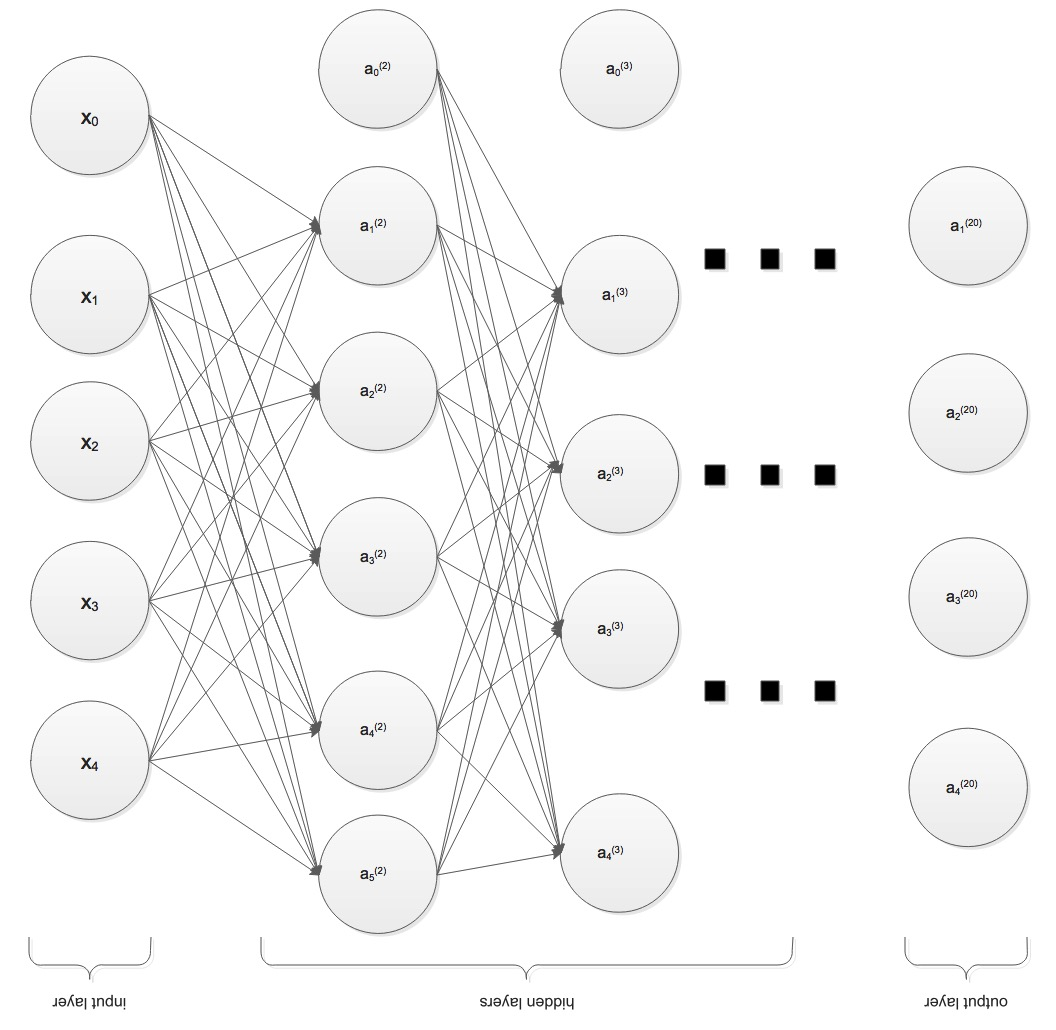### Simple Applications

This section, I’d like to construct a Neural Network to simulate a logic gate. Remember that bias $x_0$ is always $1$. Now let set $w_{10},$$w_{11} and w_{12}, and find what will h(x) become:$$w_{10}=-30\,,w_{11}=20\,,w_{12}=20\,$$x_1 x_2 z_1 a_1 0 0 -30 0 0 1 -10 0 1 0 -10 0 1 1 10 1 Here we take advantge the property of sigmoid function, g(-10)=4.5\times 10^{-5}\approx 0 and g(10)=0.99995\approx 1. From the table we have constructed an AND logic gate. It is easy to construct an OR logic gate. We just set:$$w_{10}=-30\,,w_{11}=50\,,w_{12}=50\,$$Then we get an OR logic gate. We can construct NOR gate as well, just set:$$w_{10}=10\,,w_{11}=-20\,,w_{12}=-20\,$$Question: can we construct a$XOR$gate?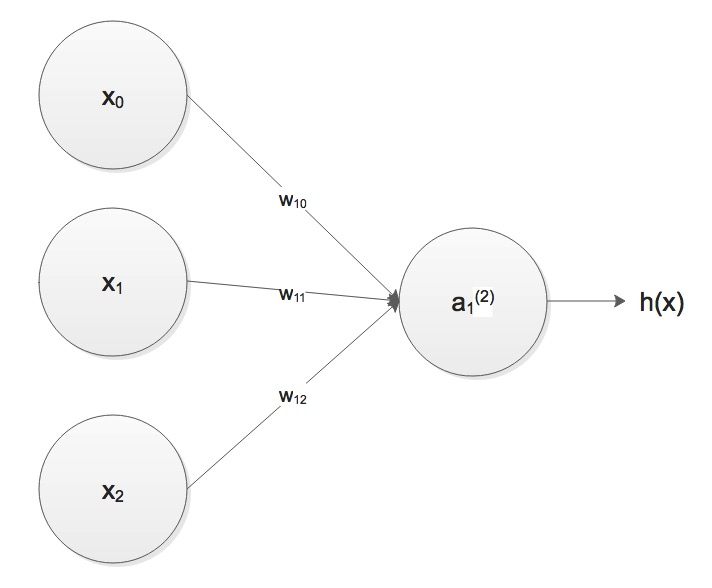In fact, we can get a more powerful logic gate through adding more hidden layers. Only 2 layers of Neural Network can not construct a$XOR$gate but 3 layers can. Neural Network shown below can implement function as$XORlogic gate.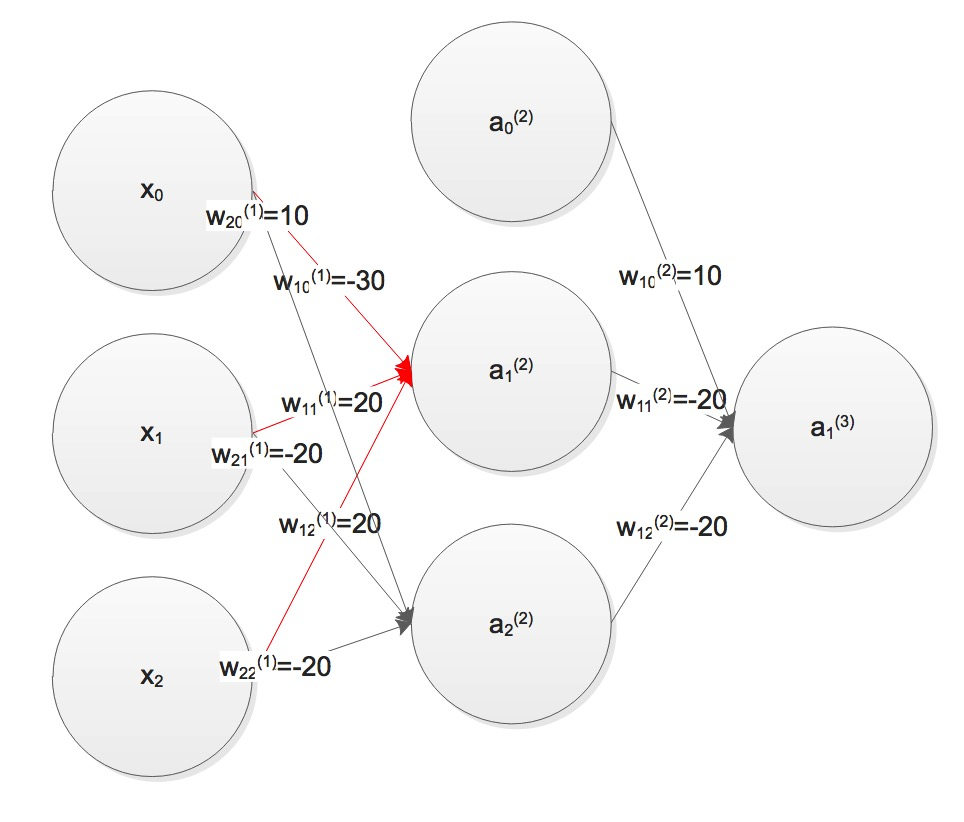The weights matrices is as followed, we can testify through table listed. \begin{align} &W^{(1)}=\begin{bmatrix}-30&20&20\ 10&-20&-20 \end{bmatrix}\ &W^{(2)}=\begin{bmatrix}10&-20&-20 \end{bmatrix} \end{align}x_1x_2a_1^{(2)}a_2^{(2)}a_1^{(3)}\$
0 0 0 1 0
0 1 0 0 1
1 0 0 0 1
1 1 1 0 0

From examples we have seen, hope you can gain intuition about Neural Network. We can generate more abstract features through adding hidden layers.

### Summerize

Today we used Logistic Regression adding hidden layers to generate Neural Network. Then we talked about how to represent a Neural Network. In the end, we found that Neural Network can simulate logic gate. We do not talk about how to train a Neural Network here. Usually we use Backpropagation Algorithm to train a Neural Network.

### Reference

1. https://www.coursera.org/learn/machine-learning
2. 《Neural Networks》by Raul Rojas